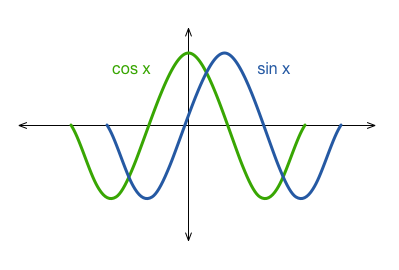# How Are The Fundamental Periods Related?

Geometry Level 2The fundamental period of $\sin x$ is $2 \pi$.
The fundamental period of $\cos x$ is $2 \pi$.

What is the fundamental period of $\sin x \cos x$?

×